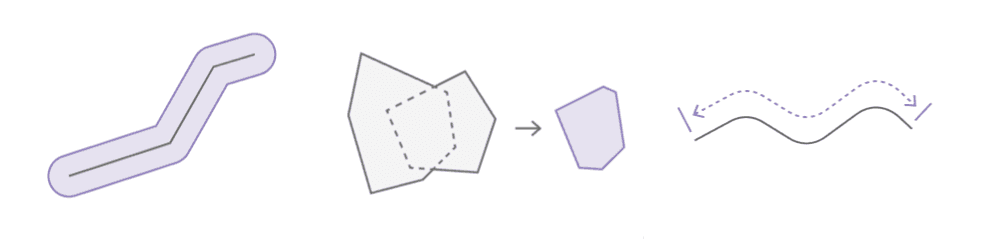# Introduction to geometry analysis## What is geometry analysis?

Geometry analysis is the process of using a client-side API to perform one or more operations on a point, polyline, or polygon to solve a geometric problem. You can use geometry operations to determine the spatial relationship between geometries, perform calculations such as creating a buffer, measure distance and area, and project geometries. The operation you use depends on the type of problem you are trying to solve.

## Types of geometry operations

Geometry operations are grouped based on the type of calculation they perform and value returned.

CategoryDescriptionOperationsResult
Example
Spatial relationshipDetermine the relationship between geometries.`contains`, `disjoint`, `intersects`, `overlaps`, `touches`, `within``boolean`Determine the intersection of two geometries with `intersects`.
Geometric calculationCalculate and create new geometries from an existing geometries.`boundary`, `buffer`, `clip`, `convexHull`, `cut`, `densify`, `difference`, `extent`, `generalize`, `intersect`, `nearestCoordinate`, `nearestVertex`, `offset`, `relate`, `reshape`, `simplify`, `trim`, `union``geometry`Buffer a line at 10 meters with `buffer`.
Length and areaMeasure length, area, or distance.`area`, `distance`, `length``number`Calculate the geodesic length of a line with `length`.
ProjectionProject to a new spatial reference.`project``geometry`Project a geometry to a new spatial reference with `project`.